In the verge of coronavirus pandemic, we are providing FREE access to our entire Online Curriculum to ensure Learning Doesn't STOP!

# Cartesian System

Go back to  'Coordinate-Geometry'

The system which we have described to label points in a plane is known as the Cartesian System. It was first described by a French mathematician named Rene Descartes in the 17th century. The word Cartesian is derived from Descartes.

• We have seen that two perpendicular number lines X and Y are required for the Cartesian System.

• The two lines X and Y taken together are called the axes of the system. Note that “axes” is a plural word. If we have to refer to just one of the two number lines X or Y, we will have to use the singular axis.

• The intersection point of the axes is the zero of the Cartesian System. This point will generally be denoted by O.

• To specify the position of any point P in the plane, we measure the distance x we have to travel along X, and then the distance y we have to travel parallel to Y, to reach from O to P. Distances can be negative. For example, if you have to travel right, then x will be positive. Similarly, if you have to travel down on Y, then y will be negative.

• The two real numbers x and y taken together will describe the position of P uniquely. We can write this as follows: P ≡ (x, y) or P = (x, y). Thus, the location of P can be labeled uniquely by two real numbers.

• For different positions of P, this pair of real numbers will be different.

• Consider the following figure and reread the discussion above once again with reference to this: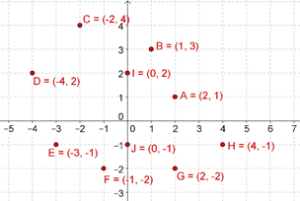• The pair of real numbers x and y which uniquely determine the position of a point P in the plane are referred to collectively as the coordinates of P.

• The first real number (in this case, x) tells us the distance we will have to cover along X to reach P. We will refer to this real number as the x-coordinate or abscissa of P.

• The second real number (in this case, y) tells us the distance we will have to cover parallel to Y to reach P. We will refer to this real number as the y-coordinate or ordinate of P.

• The x-coordinate and y-coordinate can be positive, zero or negative, depending on the location of P.

The two axes (X and Y) divide the plane into four regions, or quadrants. These are numbered as follows: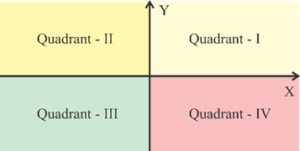In each quadrant, the signs of the coordinates will be different:

• First quadrant: x $$>$$ 0, y $$>$$ 0

• Second quadrant: x $$<$$ 0, y $$>$$ 0

• Third quadrant: x $$<$$ 0, y $$<$$ 0

• Fourth quadrant: x $$>$$ 0, y $$<$$ 0

Can you see why this is true? We also note the following:

• Points on the X axis have a y-coordinate of 0.

• Points on the Y axis have an x-coordinate of 0.

The most powerful property of the Cartesian System is that using this, any point in the plane can be uniquely labeled:

• One Dimension. On a line, points can be labeled using just one real number.

• Two Dimensions. In a plane, points can be labeled through the Cartesian system using two real numbers, or coordinates.

Suppose that you are asked to plot the point P = (2,3) in the plane. How will you do it? Starting from O (the origin), you will move 2 units to the right, along the X-axis, and then 3 units up, parallel to the Y-axis. The point you reach will be the point P: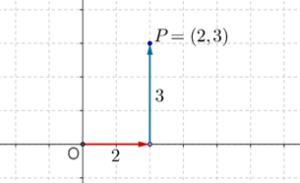Now, suppose that you have to plot Q( - 3, - 2.5). To reach Q (starting from O), you will move 3 units to the left of O, and then 2.5 units down, parallel to the Y-axis. The point you reach will be the point Q (note that Q is in the third quadrant):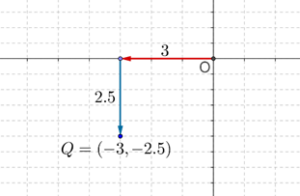This way, you can plot any point in the plane, given its coordinates.

Example 1: Plot the following points in the Cartesian plane:

•
1.  A (1.3, 2.4)
2.  B ( - 2.7, 3.2)
3.  C ( - 1.1, - 3.6)
4.  D (4, - 2)

Solution: We note that A, B, C and D are respectively in the first, second, third and fourth quadrants: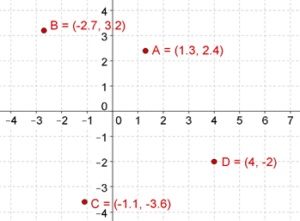Example 2: A number of points are given to you in the plane:

•
1.  A (1,4)
2.  B ( - 3, 5)
3.  C (2, - 5)
4.  D ( - 1, - 4)
5.  E (4, 4)
6.  F (1,0)
7.  G (0, - 3)

a) Which points lie in either the first or the third quadrants?

b) Which points lie in either the second of the fourth quadrants?

c) Which points lie on one of the two axes?

Solution: All the seven points have been plotted below; observe this plot carefully: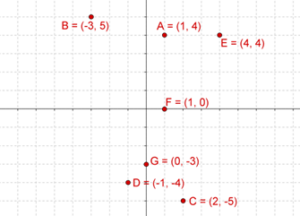a) A and E lie in the first quadrant, and D lies in the third quadrant.

b) B lies in the second quadrant, and C lies in the fourth quadrant.

c) F lies on the positive side of the X-axis, and G lies on the negative side of the Y-axis.

Example 3:A person rolls two rolling dice at the same time. Let the numbers which show on Die - 1 and Die - 2 be represented by x and y respectively. After each roll, the point P(x, y) is plotted in the plane. Plot all the possible positions of P, and highlight those positions for which the sum of x and y is 8.

Solution: Note that on each die, we can have 6 numbers (integers from 1 to 6). Thus, if you combine the possible numbers from both dice, you have 36 pairs. For example, there are six pairs with the number 3 on Die - 1:

(3,1), (3,2), (3,3), (3,4), (3,5), (3,6)

In all, there will be 36 pairs (list them out if you are unsure about this). Now, the pairs for which the sum of x and y is 8, are:

(2,6), (3,5), (4,4), (5,3), (6,2)

In the following figure, the 36 total pairs have been plotted, and these 5 pairs have been highlighted: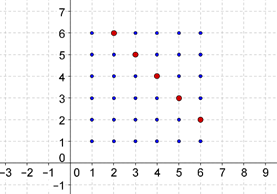Example 4: Plot five points in the Cartesian plane for which the abscissa and ordinate are equal.

Solution: We will plot the following five points:

( - 2, - 2), ( - 1, - 1), (0, 0), (1,1), (2,2)

The plot is shown below: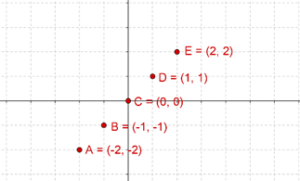Note an interesting fact. These five points lie on the same straight line: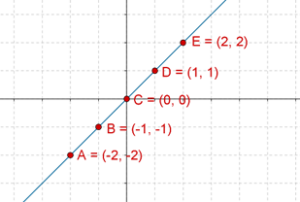In fact, if you are to take any point on this line, its abscissa and ordinate will turn out to be equal! Try it. Can you intuitively justify why this should be so?

Coordinate Geometry
Grade 9 | Questions Set 1
Coordinate Geometry
Coordinate Geometry
Grade 10 | Questions Set 1
Coordinate Geometry